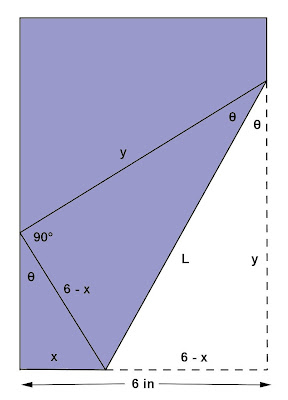## Friday, March 29, 2013

### Proving Trigonometric Identities, 3

Category: Trigonometry, Algebra

"Published in Suisun City, California, USA"

The lower right-hand corner of a long piece of paper 6 in. wide is folded over to the left-hand edge as shown. The length L of the fold depends on the angle θ. Show thatPhoto by Math Principles in Everyday Life

Solution:

Consider the given figure above and label further using the principles of Plane Geometry, we havePhoto by Math Principles in Everyday Life

By Pythagorean Theorem,

We need to use another equations in order to eliminate x and y. By using trigonometric functions of right triangles, we have

Using a small right triangle

Using a large right triangle

Consider again the equation,

Substitute the values of x and y, we have

Take the square root on both sides of the equation, we have

Multiply both the numerator and the denominator by 1 - sin2 θ, we have

Therefore,

## Thursday, March 28, 2013

### Logarithmic Expression

Category: Algebra

"Published in Suisun City, California, USA"

Rewrite the expression for

Solution:

Consider the given equation above

Expand the logarithmic expression for the main fraction, as follows

Expand the logarithmic expression for the second term, as follows

Rewrite the logarithmic expression for the square root and cube root functions and therefore,